# MUTUAL MEAN-TEACHING|PSEUDO LABEL REFINERY FOR UNSUPERVISED DOMAIN ADAPTATION ON PERSON RE-IDENTIFICATION

## Unsupervised Domain Adaptation Person Re-ID

Posted by JoselynZhao on July 7, 2020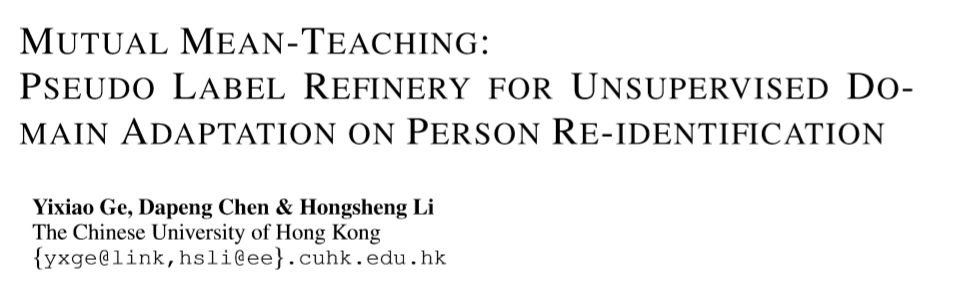为了减轻噪音伪标签的影响，文章提出了一种无监督的MMT（Mutual Mean-Teaching）方法，通过在迭代训练的方式中使用离线精炼硬伪标签和在线精炼软伪标签，来学习更佳的目标域中的特征。同时，还提出了可以让Traplet loss支持软标签的soft softmax-triplet loss”。 该方法在域自适应任务方面明显优于所有现有的Person re-ID方法，改进幅度高达18.2％。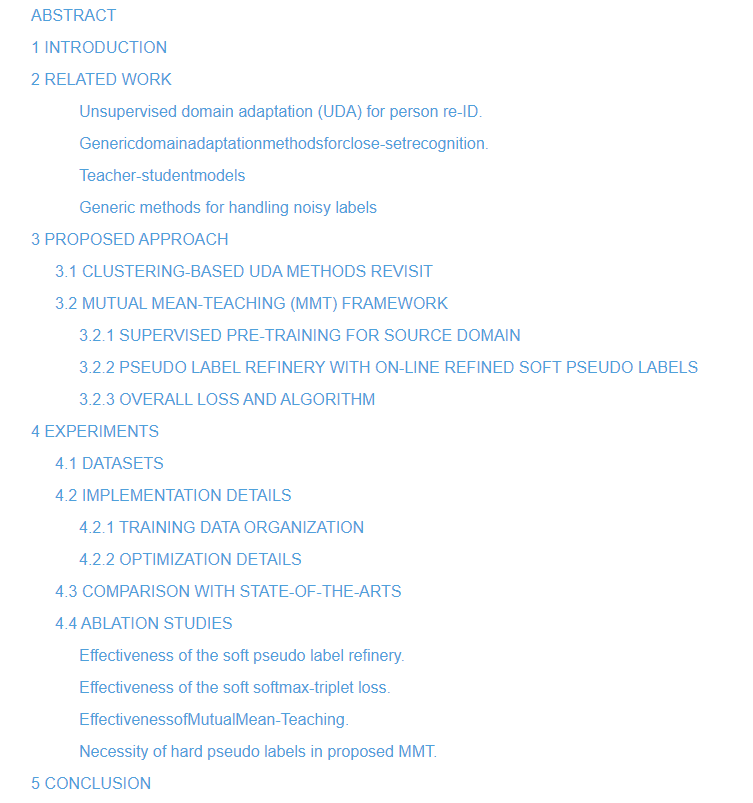# ABSTRACT

Do What In order to mitigate the effects of noisy pseudo labels:

• we propose to softly reﬁne the pseudo labels in the target domain by proposing an unsupervised framework, Mutual Mean-Teaching (MMT), to learn better features from the target domain via off-line reﬁned hard pseudo labels and on-line reﬁned soft pseudo labels in an alternative training manner.
• the common practice is to adopt both the classiﬁcation loss and the triplet loss jointly for achieving optimal performances in person re-ID models. However, conventional triplet loss cannot work with softly reﬁned labels. To solve this problem, a novel soft softmax-triplet loss is proposed to support learning with soft pseudo triplet labels for achieving the optimal domain adaptation performance.

Results： The proposed MMT framework achieves considerable improvements of 14.4%, 18.2%, 13.4% and 16.4% mAP on Market-to-Duke, Duke-to-Market, Market-to-MSMT and Duke-to-MSMT unsupervised domain adaptation tasks.

# 1 INTRODUCTION

State-of-the-art UDA methods (Song et al., 2018; Zhang et al., 2019b; Yang et al., 2019) for person re-ID group unannotated images with clustering algorithms and train the network with clustering-generated pseudo labels.

Conclusion 1 The reﬁnery of noisy pseudo labels has crucial inﬂuences to the ﬁnal performance, but is mostly ignored by the clustering-based UDA methods.

To effectively address the problem of noisy pseudo labels in clustering-based UDA methods (Song et al., 2018; Zhang et al., 2019b; Yang et al., 2019) (Figure 1), we propose an unsupervised Mutual Mean-Teaching (MMT) framework to effectively perform pseudo label reﬁnery by optimizing the neural networks under the joint supervisions of off-line reﬁned hard pseudo labels and on-line reﬁned soft pseudo labels.

Speciﬁcally, our proposed MMT framework provides robust soft pseudo labels in an on-line peer-teaching manner, which is inspired by the teacher-student approaches (Tarvainen & Valpola, 2017; Zhang et al., 2018b) to simultaneously train two same networks. The networks gradually capture target-domain data distributions and thus reﬁne pseudo labels for better feature learning. To avoid training error ampliﬁcation, the temporally average model of each network is proposed to produce reliable soft labels for supervising the other network in a collaborative training strategy. By training peer-networks with such on-line soft pseudo labels on the target domain, the learned feature representations can be iteratively improved to provide more accurate soft pseudo labels, which, in turn, further improves the discriminativeness of learned feature representations.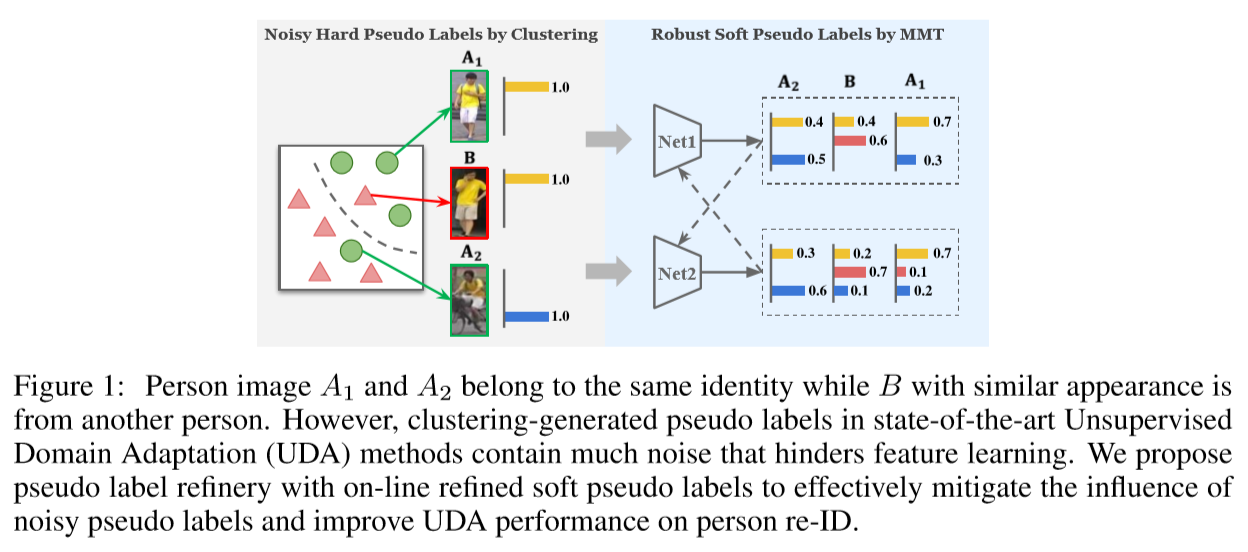the conventional triplet loss (Hermansetal.,2017)cannot work with such reﬁned soft labels. To enable using the triplet loss with soft pseudo labels in our MMT framework, we propose a novel soft softmax-triplet loss so that the network can beneﬁt from softly reﬁned triplet labels.

The introduction of such soft softmax-triplet loss is also the key to the superior performance of our proposed framework. Note that the collaborative training strategy on the two networks is only adopted in the training process. Only one network is kept in the inference stage without requiring any additional computational or memory cost.

The contributions of this paper could be summarized as three-fold.

• The proposed Mutual Mean-Teaching (MMT) framework is designed to provide more reliable soft labels.
• we propose the soft softmax-triplet loss to learn more discriminative person features.
• The MMT framework shows exceptionally strong performances on all UDA tasks of person re-ID.

# 3 PROPOSED APPROACH

Our key idea is to conduct pseudo label reﬁnery in the target domain by optimizing the neural networks with off-line reﬁned hard pseudo labels and on-line reﬁned soft pseudo labels in a collaborative training manner.

In addition, the conventional triplet loss cannot properly work with soft labels. A novel soft softmax-triplet loss is therefore introduced to better utilize the softly reﬁned pseudo labels.

Both the soft classiﬁcation loss and the soft softmax-triplet loss work jointly to achieve optimal domain adaptation performances.

Formally:

we denote the source domain data as $D_s = \left\{(x^s_i,y^s_i )\|^{N_s}_{i=1}\right\}$, where $x^s_i$and $y^s_i$ denote the i-th training sample and its associated person identity label, $N_s$ is the number of images, and $M_s$ denotes the number of person identities (classes) in the source domain. The $N_t$ target-domain images are denoted as $Dt = \left\{x^t_i\|^{N_t}_{i=1}\right\}$, which are not associated with any ground-truth identity label.

## 3.1 CLUSTERING-BASED UDA METHODS REVISIT

State-of-the-art UDA methods generally pre-train a deep neuralnet work $F(·\|θ)$onthe source domain, where $θ$ denotes current network parameters, and the network is then transferred to learn from the images in the target domain.

The source-domain images’ and target-domain images’ features encoded by the network are denoted as $\left\{F(x^s_i\|θ)\right\}\|^{N_s}_{ i=1}$ and $\left\{F(x^t_i\|θ)\right\}\|^{N_t}_{ i=1}$ respectively.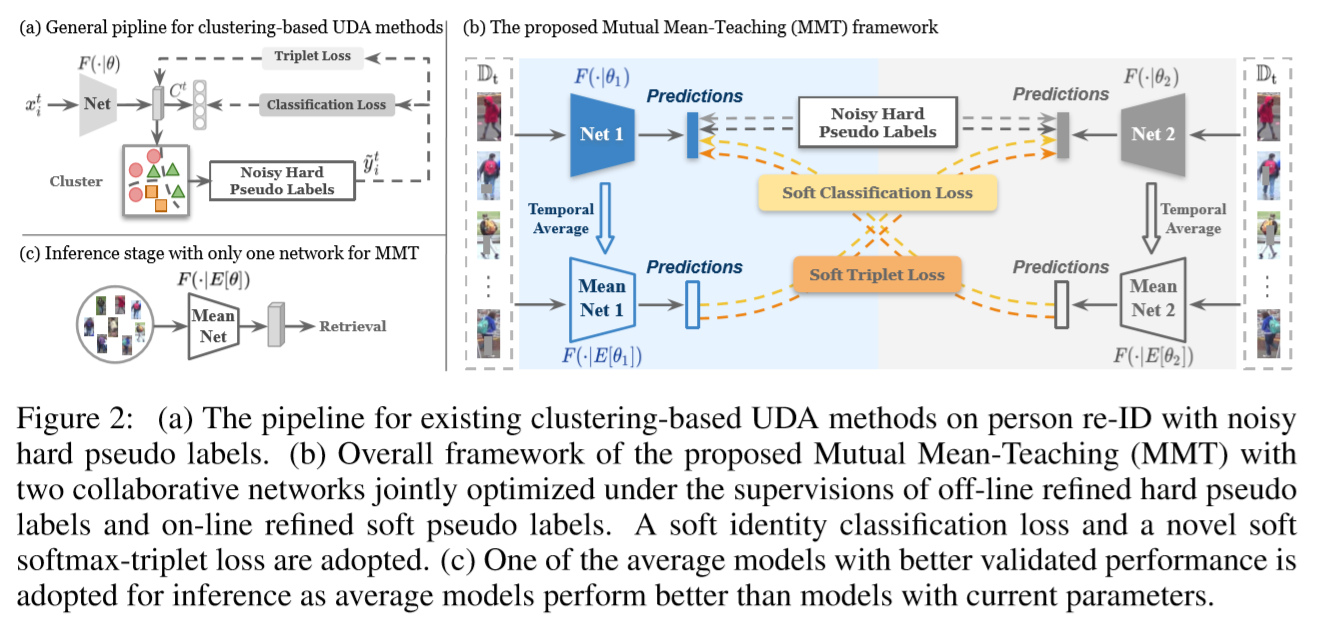As illustrated in Figure 2 (a), two operations are alternated to gradually ﬁne-tune the pre-trained network on the target domain.

• The target-domain samples are grouped into pre-deﬁned $M_t$classes by clustering the features $\left\{F(x^t_i\|θ)\right\}\|^{N_t}_{ i=1}$ output by the current network. Let $\hat{h^t_i}$ denotes the pseudo label generated for image $x^t_i$.
• The network parameters $θ$ and a learnable target-domain classiﬁer $C^t : f^t →\left\{1,··· ,M_t\right\}$ are then optimized with respect to an identity classiﬁcation(crossentropy) loss $L^t_{id}(θ)$ and a triplet loss (Hermans et al., 2017) $L^t_{tri}(θ)$ in the form of,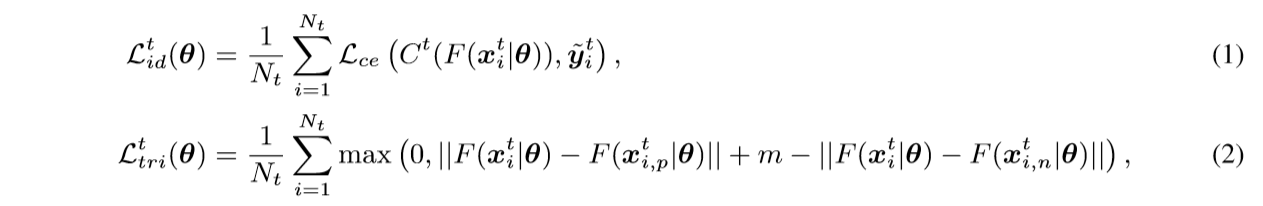where ||·|| denotes the L2-norm distance, subscripts i,p and i,n indicate the hardest positive and hardest negative feature index in each mini-batch for the sample $x^t_i$, and m = 0.5 denotes the triplet distance margin.

## 3.2 MUTUAL MEAN-TEACHING (MMT) FRAMEWORK

### 3.2.1 SUPERVISED PRE-TRAINING FOR SOURCE DOMAIN

The neural network is trained with a classiﬁcation loss $L^s_{id}(θ)$ and a triplet loss $L^s_{tri}(θ)$ to separate features belonging to different identities. The overall loss is therefore calculated as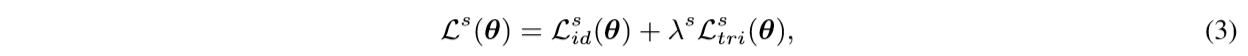where $\lambda^s$ is the parameter weighting the two losses.

### 3.2.2 PSEUDO LABEL REFINERY WITH ON-LINE REFINED SOFT PSEUDO LABELS

off-linereﬁned hard pseudo labels as introduced in Section 3.1, where the pseudo label generation and reﬁnement are conducted alternatively. However, the pseudo labels generated in this way are hard (i.e.,theyare always of 100% conﬁdences) but noisy

our framework further incorporates on-line reﬁned soft pseudo labels (i.e., pseudo labels with < 100% conﬁdences) into the training process.

Our MMT framework generates soft pseudo labels by collaboratively training two same networks with different initializations. The overall framework is illustrated in Figure 2 (b).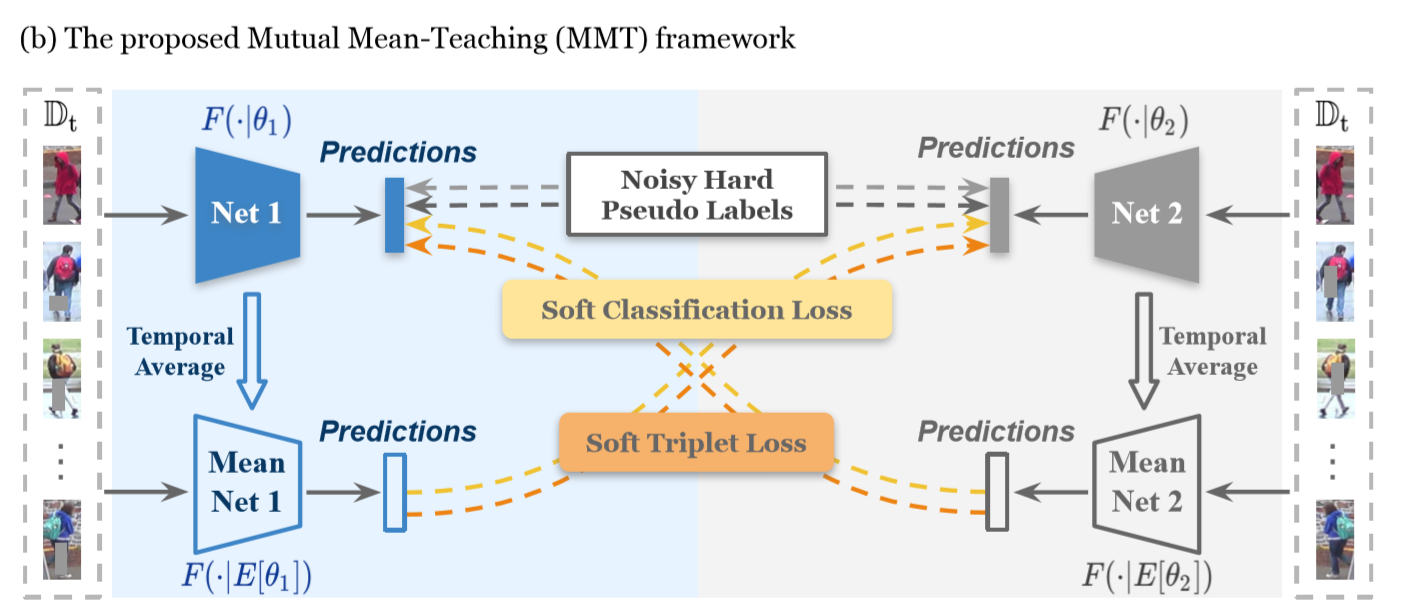our two collaborative networks also generate on-line soft pseudo labels by network predictions for training each other.

To avoid two networks collaboratively bias each other, the past temporally average model of each network instead of the current model is used to generate the soft pseudo labels for the other network. Both off-line hard pseudo labels and on-line soft pseudo labels are utilized jointly to train the two collaborative networks. After training,only one of the past average models with better validated performance is adopted for inference (see Figure 2 (c)).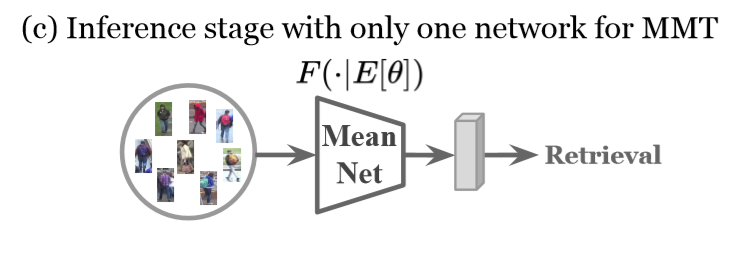We denote the two collaborative networks as feature transformation functions $F(·\|θ_1)$ and $F(·\|θ_2)$, and denote their corresponding pseudo label classiﬁers as $C^t _1$ and $C^t_2$, respectively.

we feed the same image batch to the two networks but with separately random erasing, cropping and ﬂipping.

Each target-domain image can be denoted by $x^t_i$ and $x'^t_i$ for the two networks, and their pseudo label conﬁdences can be predicted as $C^t_1(F(x^t_i\|θ_1))$ and $C^t _2(F(x'^t_i\|θ_2))$.

In order to avoid error ampliﬁcation, we propose to use the temporally average model of each network to generate reliable soft pseudo labels for supervising the other network.

Speciﬁcally, the parameters of the temporally average models of the two networks at current iteration T are denoted as $E^{(T)}[θ_1]$ and $E^{(T)}[θ_2]$ respectively, which can be calculated as :where $E^{(T)}[θ_1]$, $E^{(T)}[θ_2]$ indicate the temporal average parameters of the two networks in the previous iteration(T−1), the initial temporal average parameters are $E^{(0)}[θ_1] = θ_1$, $E^{(0)}[θ_2] = θ2$,and α is the ensembling momentum to be within the range [0,1).

The robust soft pseudo label supervisions are then generated by the two temporal average models as $C^t_1(F(x^t_i\|E^{(T)}[θ_1]))$ and $C^t_2(F(x^t_i\|E^{(T)}[θ_2]))$ respectively. The soft classiﬁcation loss for optimizing $θ_1$ and $θ_2$ with the soft pseudo labels generated from the other network can therefore be formulated as: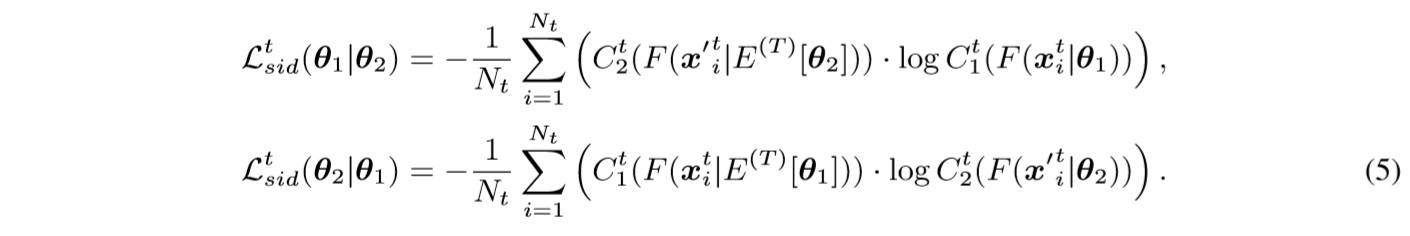The two networks’ pseudo-label predictions are better dis-related by using other network’s past average model to generate supervisions and can therefore better avoid error ampliﬁcation.

we propose to use softmax-triplet loss, whose hard version is formulated as：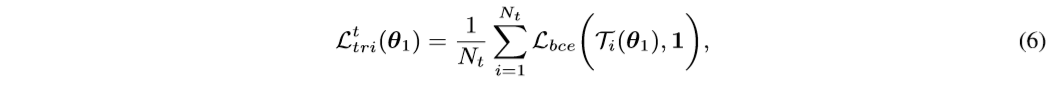where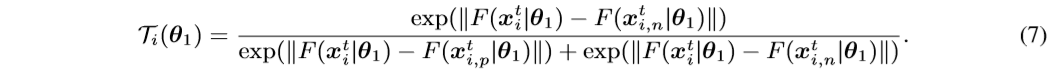Here $L_{bce}(·,·)$ denotes the binary cross-entropy loss. “1” denotes the ground-truth that the positive sample $x^t_{i,p}$ should be closer to the sample $x^t_i$ than its negative sample $x^t_{i,n}$.

we can utilize the one network’s past temporal average model to generate soft triplet labels for the other network with the proposed soft softmax-triplet loss: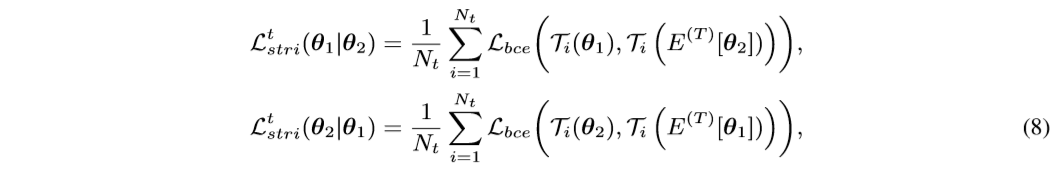where $T_i(E^{(T)}[θ_1])$ and $T_i(E^{(T)}[θ_2])$ are the soft triplet labels generated by the two networks’ past temporally average models.

### 3.2.3 OVERALL LOSS AND ALGORITHM

Our proposed MMT framework is trained with both off-line reﬁned hard pseudo labels and on-line reﬁnedsoftpseudolabels. The over all loss function $L(θ_1,θ_2)$ simultaneously optimizes the coupled networks, which combines equation 1, equation 5, equation 6, equation 8 and is formulated as,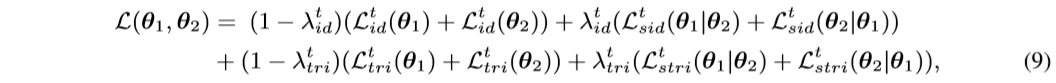where $λ^t_{id}$, $λ^t_{tri}$ aretheweightingparameters.

The detailed optimization procedures are summarized in Algorithm 1.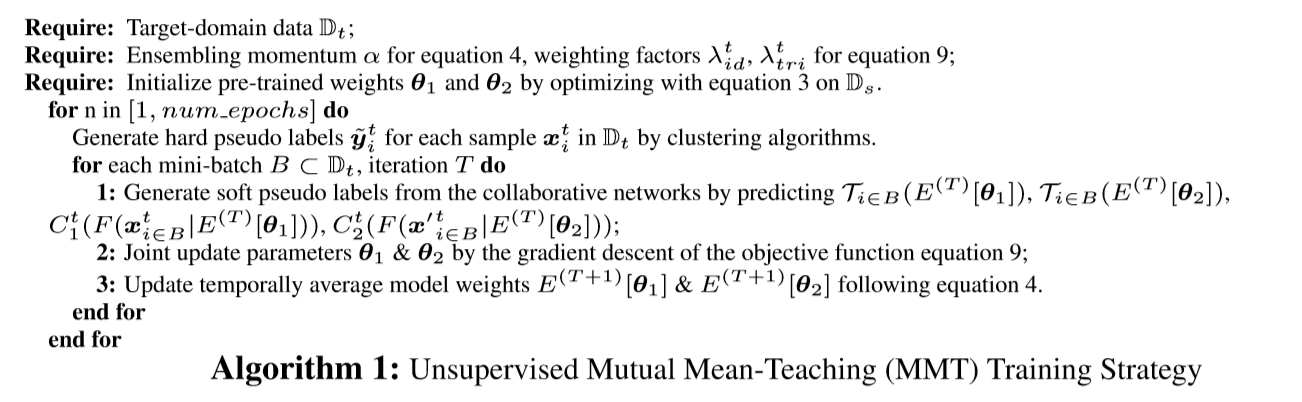The hard pseudo labels are off-line reﬁned after training with existing hard pseudo labelsforoneepoch. During the training process, the two networks are trained by combining the off line reﬁned hard pseudo labels and on-line reﬁned soft labels predicted by their peers with proposed soft losses. The noise and randomness caused by hard clustering, which lead to unstable training and limited ﬁnal performance, can be alleviated by the proposed MMT framework.

# 4 EXPERIMENTS

## 4.1 DATASETS

We evaluate our proposed MMT on three widely-used person re-ID datasets, i.e., Market1501(Zhengetal.,2015),DukeMTMC-reID(Ristanietal.,2016),andMSMT17(Weietal.,2018).

For evaluating the domain adaptation performance of different methods, four domain adaptation tasks are set up, i.e., Duke-to-Market, Market-to-Duke, Duke-to-MSMT and Market-to-MSMT, where only identity labels on the source domain are provided. Mean average precision (mAP) and CMC top-1, top-5, top-10 accuracies are adopted to evaluate the methods’ performances.

## 4.2 IMPLEMENTATION DETAILS

### 4.2.1 TRAINING DATA ORGANIZATION

For both source-domain pre-training and target-domain ﬁne-tuning, each training mini-batch contains 64 person images of 16 actual or pseudo identities (4 for each identity). All images are resized to 256×128 before being fed into the networks.

### 4.2.2 OPTIMIZATION DETAILS

All the hyper-parameters of the proposed MMT framework are chosen based on a validation set of the Duke-to-Market task with $M_t$ = 500 pseudo identities and IBN-ResNet-50 backbone.

We propose a two-stage training scheme, where ADAM optimizer is adopted to optimize the networks with a weight decay of 0.0005. Randomly erasing (Zhong et al., 2017b) is only adopted in target-domain ﬁne-tuning.

• Stage 1: Source-domain pre-training.
• Stage 2: End-to-end training with MMT.

## 4.3 COMPARISON WITH STATE-OF-THE-ARTS

The results are shown in Table 1. Our MMT framework signiﬁcantly outperforms all existing approaches with both ResNet-50 and IBN-ResNet-50 backbones, which veriﬁes the effectiveness of our method.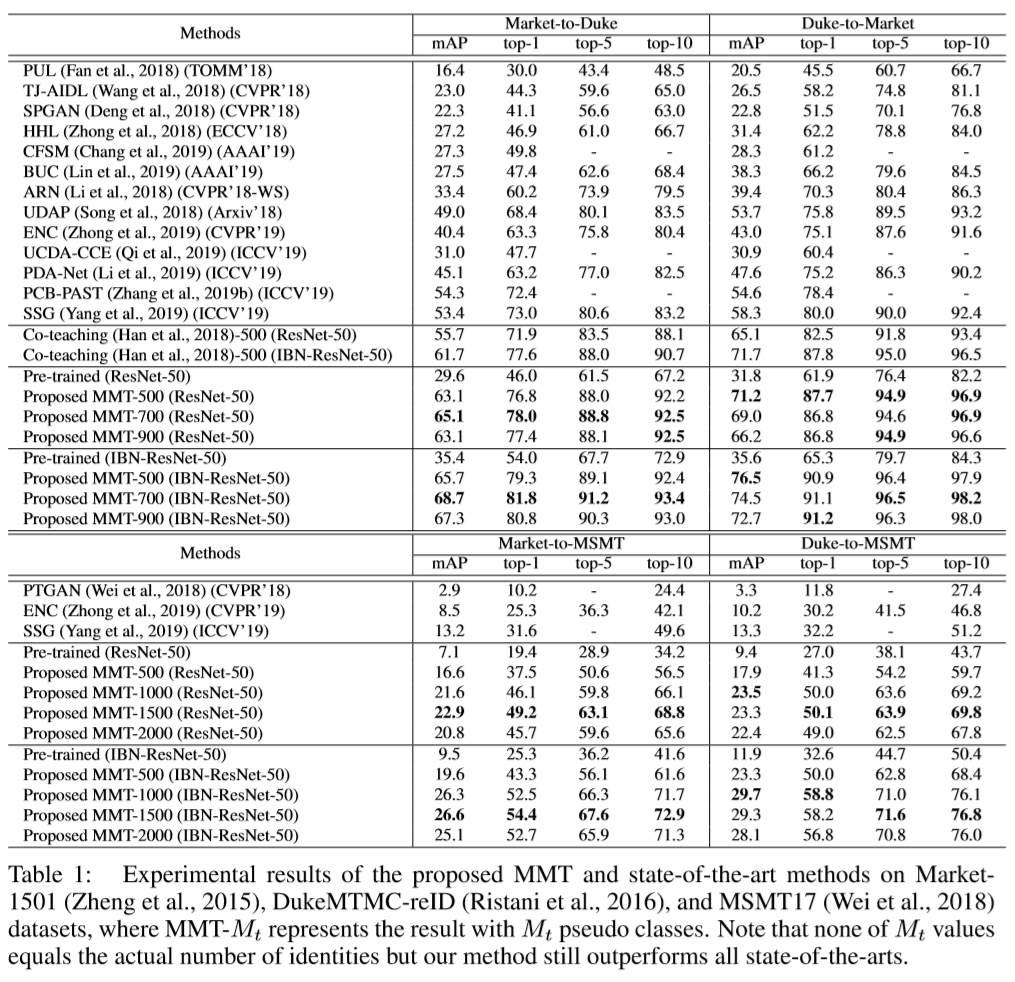Such results prove the necessity and effectiveness of our proposed pseudo label reﬁnery for hard pseudo labels with inevitable noises.

Co-teaching (Han et al., 2018) is designed for general close-set recognition problems with manually generated label noise, which could not tackle the real-world challenges in unsupervised person re-ID. More importantly, it does not explore how to mitigate the label noise for the triplet loss as our method does.

## 4.4 ABLATION STUDIES

In this section, we evaluate each component in our proposed framework by conducting ablation studies on Duke-to-Market and Market-to-Duke tasks with both ResNet-50 (He et al., 2016) and IBN-ResNet-50 (Pan et al., 2018) backbones. Results are shown in Table 2.### Effectiveness of the soft pseudo label reﬁnery.

To investigate the necessity of handling noisy pseudo labels in clustering-based UDA methods,we create baseline models that utilize only off-line reﬁned hard pseudo labels. i.e., optimizing equation 9 with $λ^t_{id} = λ^t_{tri} = 0$ for the two-step training strategy in Section 3.1. The baseline model performances are present in Table 2 as “Baseline (only $L^t_{id}$ &$L^t_{tri}$)”.

### Effectiveness of the soft softmax-triplet loss.

We also verify the effectiveness of soft softmaxtriplet loss with softly reﬁned triplet labels in our proposed MMT framework. Experiments of removing the soft softmax-triplet loss, i.e., $λ^t_{tri} = 0$ in equation 9, but keeping the hard softmax-triplet loss (equation 6) are conducted, which are denoted as “Baseline+MMT-500 (w/o Ltstri)”.

### EffectivenessofMutualMean-Teaching.

We propose to generate on-line reﬁned soft pseudo labels for one network with the predictions of the past average model of the other network in our MMT framework ,i.e., the soft labels for network1 are output from the average model of network2 an dvice versa.

### Necessity of hard pseudo labels in proposed MMT.

To investigate the contribution of $L^t_{id}$ in the ﬁnal training objective function as equation 9, we conduct two experiments.

• (1) “Baseline+MMT-500 (only $L^t_{sid}$ & $L^t_{stri}$)” by removing both hard classiﬁcation loss and hard triplet loss with $λ^t_{id} = λ^t_{tri} = 1$;
• (2)“Baseline+MMT-500 (w/o $L^t_{id})$” by removing only hard classiﬁcation loss with $λ^t_{id} = 1$.

# 5 CONCLUSION

In this work, we propose an unsupervised Mutual Mean-Teaching (MMT) framework to tackle the problem of noisy pseudo labels in clustering-based unsupervised domain adaptation methods for person re-ID. The key is to conduct pseudo label reﬁnery to better model inter-sample relations in the target domain by optimizing with the off-line reﬁned hard pseudo labels and on-line reﬁned soft pseudo labels in a collaborative training manner. Moreover, a novel soft softmax-triplet loss is proposed to support learning with softly reﬁned triplet labels for optimal performances. Our method signiﬁcantly outperforms all existing person re-ID methods on domain adaptation task with up to 18.2% improvements.